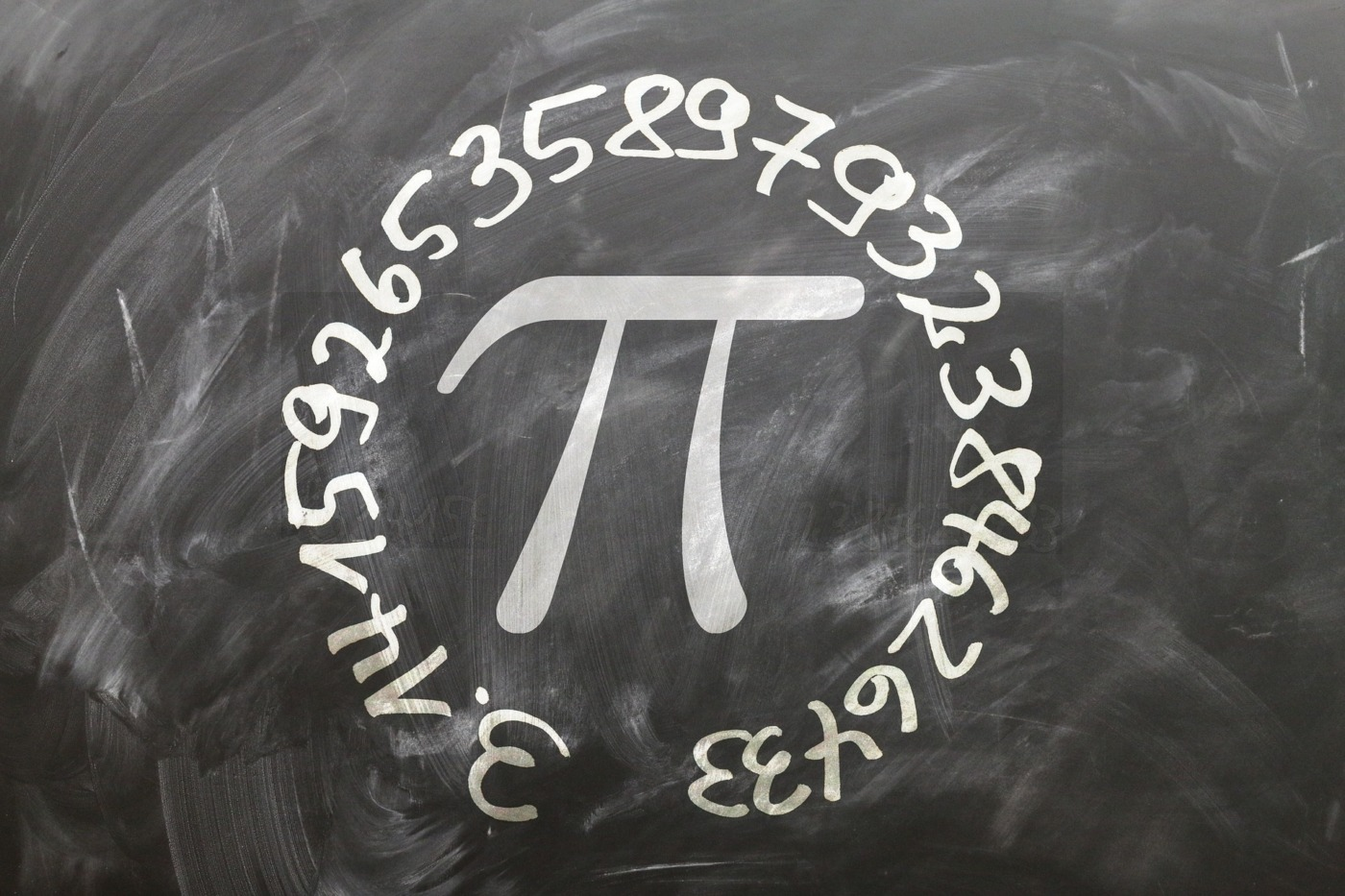# Swiss researchers calculate pi to a record 62.8 trillion digits

Pi (π) is a number that everyone is broadly familiar with – if you did maths at school, no doubt the value of 3.14 was drilled into you. But it’s hard to conceptualise just how long the number is. The previous world record pi calculation achieved 50 trillion digits, but now a team of Swiss researchers have claimed a new world record for calculating the digits of pi – an incredible 62.8 trillion figures. Given that we only need 65 decimal places to know the size of the observable universe to within a Planck length (the shortest possible measurable distance), one question to ask is why bother at all. The digits may not be scientifically useful, but there are lots of reasons that mathematicians and computer scientists are awaiting the details of this calculation.

What makes pi special? It’s an irrational number, meaning that it needs infinitely many decimals to express it, but it’s also an irrational number with no repeating patterns. If you wanted to express a number like 1/7 decimally, you could write 0.1428571428571… and so on, but that’s not possible with pi. It is also something known as a transcendental number, meaning it cannot be defined through any simple equation featuring whole numbers, something known as early as 1768. And, of course, there’s its links with circles – pi is the ratio of a circle’s circumference to its diameter, and that fact enables us to calculate the circumference of some incredibly large bodies, from the Earth and the Milky Way to the observable universe.

As a result, scientists throughout history have approximated and found closer values of pi. Babylonian mathematics usually set pi as 3, which was sufficient for the architectural projects of the time, although one tablet from the time offered a value of 25/8. It has been suggested that the ancient Egyptians used 22/7, while Archimedes used geometry to prove that 223/71< π<22/7 in the 3rd century BCE. Techniques changed dramatically after the 17th century, with the development of calculus and infinite series, and processes were discovered that could get closer and closer to the true value of pi. One example is the Madhava series, which says π=4(1-1/3+1/5-1/7+1/9…), but it takes a lot of terms to produce correct decimal values.

The process will help facilitate the development and testing of supercomputers and high-precision algorithms

The infinite sum used to calculate the 2020 world record of 50 trillion digits was discovered in 1988, and it can calculate 14 new digits of pi for each new term that is added to the sum. According to a statement from the Graubuenden University of Applied Sciences, the calculation of the new record “took 108 days and nine hours”, which is “almost twice as fast as the record Google set using its cloud in 2019, and 3.5 times as fast as the previous world record in 2020”, although the researchers are waiting on the Guinness Book of Records to certify the total. If the total is certified, the new number will be phenomenally long – Julia Collins, lecturer in maths at Edith Cowan University, estimates that the number would be long enough to fill every book in the British Library ten times over.

As we don’t technically need these numbers, what’s the use of this experiment? The process will help facilitate the development and testing of supercomputers and high-precision algorithms, which could lead to hardware and software that will benefit other areas of our lives – the statement cites “RNA analysis, simulations of fluid dynamics and textual analysis”. It will also allow mathematicians to further explore the nature of pi, including the analysis of whether it is a ‘normal’ number, meaning all possible sequences of digits should appear equally often. Mathematicians don’t know if each decimal digits appears infinitely often, nor if there are more complex patterns to find.

The team currently has no plans to calculate more of pi’s decimal places, and they’re confident that someone else will claim the record before long. Thomas Keller, a computer scientist at the University of Applied Sciences of the Grisons, said that: “Looking at the previous pace of record setting, I anticipate the next successful record-breaking attempt any time in the space of the next two years.”

[related_posts_by_tax columns="4" posts_per_page="4" format="thumbnails" image_size="medium" exclude_terms="34573"]